# Test: Chemical Kinetics - 1

## 25 Questions MCQ Test Topic-wise MCQ Tests for NEET | Test: Chemical Kinetics - 1

Description
Attempt Test: Chemical Kinetics - 1 | 25 questions in 35 minutes | Mock test for NEET preparation | Free important questions MCQ to study Topic-wise MCQ Tests for NEET for NEET Exam | Download free PDF with solutions
QUESTION: 1

### Which of the following statement is true for order of a reaction?

Solution:

Order of a reaction is experimentally determined quantity so it can be negative fractional or zero.

QUESTION: 2

### For the first order reaction, half life is equal to

Solution:

For 1st order reaction half life is independent of initial concentration of reactant.

QUESTION: 3

### Straight line graph for first order reaction is obtained between

Solution: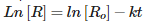QUESTION: 4

The effect of temperature on reaction rate is given by

Solution:

k = A exp(-kt)

QUESTION: 5

The chemical reaction in which reactants require high amount of activation energy are generally

Solution:

If activation energy is high rate of reaction will decrease and reaction will be slow.

QUESTION: 6

The minimum amount of energy required by the reacting molecules at the time of collisions in order to produce effective collisions is called

Solution:
QUESTION: 7

The expression which relates the rate of reaction to the concentration of the reactants are called?

Solution:

Rate law states that rate of reaction is dependent on concentration of reactant raise to power order of reaction.

QUESTION: 8

The constant k used in rate equation is known as

Solution:

Rate constant is also known as velocity constant.

QUESTION: 9

The radioactive isotope used in determining the age of organic substances is

Solution:

Carbon – 14 is used in determining the age of organic substances

QUESTION: 10

The substance that slows down the reaction without being consumed is known as

Solution:

Inhibitors inhibits the action of catalyst and hence slows down the rate of reaction.

QUESTION: 11

Which among the following statement is not true for catalyst?

Solution:

Catalyst doesnot change the equilibrium constant it only helps in reaching the equilibrium at a faster rate.

QUESTION: 12

A first order reaction is 50% completed in 1.26 × 1014 s. How much time would it take for 100% completion?

Solution:

The time taken for half the reaction to complete, i.e., the time in which the concentration of a reactant is reduced to half of its original value is called half-life period of the reaction. But it is impossible to perform 100% of the reaction. Whole of the substance never reacts because in every half-life, 50% of the substance reacts. Hence, time taken for 100% completion of a reaction is infinite.

QUESTION: 13

Collision theory is applicable to

Solution:

Collision theory is only applicable to bimolecular reaction.

QUESTION: 14

Rate of reaction does not remain constant throughout because

Solution:

Rate of reaction is dependent on concentration of reactant

QUESTION: 15

The unit of rate constant for a first order reaction is

Solution:

The correct answer is Option C.

Let R be the rate of reaction.
For first order reaction,
R=K[A]1
⇒K=R[A]-1
Whereas, K and [A] are rate constant and initial concentration of reactant respectively.
Therefore,
Unit of rate constant =(mol L-1)1-nsec-1
For first order reaction, n=1
Unit of rate constant = sec-1
Hence the unit of rate constant for first order reaction is sec-1.

QUESTION: 16

Reaction which takes place in one step is known as

Solution:

Elementary reaction are one step reactions.

QUESTION: 17

Which among the following statement is not true for rate constant of a reaction?

Solution:

Rate constant is independent of concentration of reactant.

QUESTION: 18

Which among the following is an example of pseudo first order reaction?

Solution:

In acid catalysed hydrolysis of ethylacetate the water is in excess so it is pseudo 1st order reaction.

QUESTION: 19

Which among the following is an example of first order reaction?

Solution:

Decomposition of N2O5 is 1st order reaction.

QUESTION: 20

The rate law for the reaction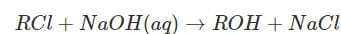is given by rate = k[RCl]. The rate for this reaction

Solution:

Since order of reaction with respect to RCl is one so if conc of RCl is halfed the rate of reaction will also become half.

QUESTION: 21

For an endothermic reaction, the minimum value for the energy of activation in terms of will be

Solution: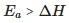QUESTION: 22

Order of the photochemical reaction occurring between hydrogen and chlorine is

Solution:

Photochemical reaction between H2 and Cl2 is 0 zero wrt to reactants.

QUESTION: 23

The temperature coefficient of most of the reactions lies between

Solution:

For every 100 rise in temperature rate of reaction increases by factor of 2 or 3.

QUESTION: 24

Following mechanism has been proposed for a reaction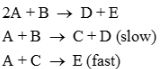The rate law expression for the reaction is

Solution:

Rate of reaction is determined by slowest step.

QUESTION: 25

The expression which gives 3/4th life of first order reaction is

Solution: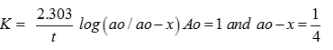Use Code STAYHOME200 and get INR 200 additional OFF Use Coupon Code